# 9.2 First Derivative for Polynomial Function

9.2 First Derivative for Polynomial Function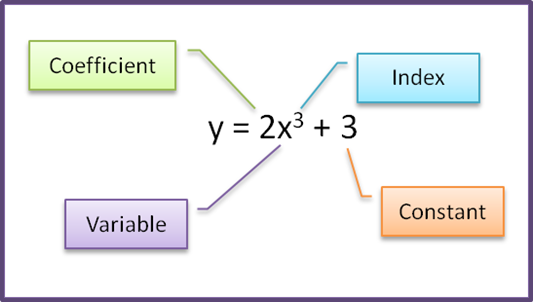(A) Differentiating a Constant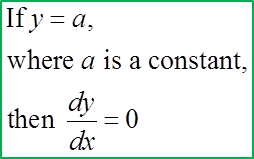(B) Differentiating Variable with Index n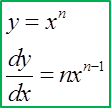(C) Differentiating a Linear Function(D) Differentiating a Polynomial Function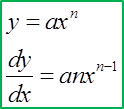(E) Differentiating Fractional Function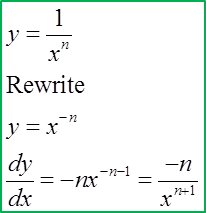(F) Differentiating Square Root FunctionExample:
Find $\frac{dy}{dx}$ for each of the following functions:
(a) y= 12
(b) y= x4
(c) y= 3x
(d) y= 5x3

Solution:
(a)
= 12
$\frac{dy}{dx}=0$

(b)
= x4
$\frac{dy}{dx}$ = 4x3

(c)
= 3x
$\frac{dy}{dx}$ = 3

(d)
= 5x3
$\frac{dy}{dx}$ = 3(5x2) = 15x2

(e)

$\begin{array}{l}y=\frac{1}{x}={x}^{-1}\\ \frac{dy}{dx}=-{x}^{-1-1}=-\frac{1}{{x}^{2}}\end{array}$

(f)

$\begin{array}{l}y=\frac{2}{{x}^{4}}=2{x}^{-4}\\ \frac{dy}{dx}=-4\left(2{x}^{-4-1}\right)=-8{x}^{-5}=-\frac{8}{{x}^{5}}\end{array}$

(g)

$\begin{array}{l}y=\frac{2}{5{x}^{2}}=\frac{2{x}^{-2}}{5}\\ \frac{dy}{dx}=-2\left(\frac{2{x}^{-2-1}}{5}\right)=-\frac{4{x}^{-3}}{5}=-\frac{4}{5{x}^{3}}\end{array}$

(h)

$\begin{array}{l}y=\text{3}\sqrt{x}=3{\left(x\right)}^{\frac{1}{2}}\\ \frac{dy}{dx}=\frac{1}{2}\left(3{x}^{\frac{1}{2}-1}\right)=\frac{3}{2}{x}^{-\frac{1}{2}}=\frac{3}{2\sqrt{x}}\end{array}$

(i)
$\begin{array}{l}y=4\sqrt{{x}^{3}}=4{\left({x}^{3}\right)}^{\frac{1}{2}}=4{x}^{\frac{3}{2}}\\ \frac{dy}{dx}=\frac{3}{2}\left(4{x}^{\frac{3}{2}-1}\right)=6{x}^{\frac{1}{2}}=6\sqrt{x}\end{array}$